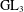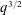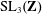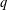Home
Hostname: page-component-cf9d5c678-dkwk2 Total loading time: 0.166 Render date: 2021-08-02T07:17:39.888Z Has data issue: true Feature Flags: { "shouldUseShareProductTool": true, "shouldUseHypothesis": true, "isUnsiloEnabled": true, "metricsAbstractViews": false, "figures": true, "newCiteModal": false, "newCitedByModal": true, "newEcommerce": true, "newUsageEvents": true }Forum of Mathematics, Sigma

# PERIODIC TWISTS OF$\operatorname{GL}_{3}$-AUTOMORPHIC FORMS

Published online by Cambridge University Press:  12 March 2020

Corresponding

## Abstract

We prove that sums of length about$q^{3/2}$ of Hecke eigenvalues of automorphic forms on$\operatorname{SL}_{3}(\mathbf{Z})$ do not correlate with$q$-periodic functions with bounded Fourier transform. This generalizes the earlier results of Munshi and Holowinsky–Nelson, corresponding to multiplicative Dirichlet characters, and applies, in particular, to trace functions of small conductor modulo primes.

## MSC classification

Type
Number Theory
Information
Creative CommonsThis is an Open Access article, distributed under the terms of the Creative Commons Attribution licence (http://creativecommons.org/licenses/by/4.0/), which permits unrestricted re-use, distribution, and reproduction in any medium, provided the original work is properly cited.

## References

Aggarwal, K., Holowinsky, R., Lin, Y. and Sun, Q., ‘The Burgess bound via a trivial delta method’, Ramanujan J. (2018), (to appear) arXiv:1803.00542.Google Scholar
Blomer, V., ‘Subconvexity for twisted L-functions on GL(3)’, Amer. J. Math. 134(5) (2012), 13851421.CrossRefGoogle Scholar
Conrey, J. B. and Iwaniec, H., ‘The cubic moment of central values of automorphic L-functions’, Ann. of Math. (2) 151(3) (2000), 11751216.CrossRefGoogle Scholar
Fouvry, É., Kowalski, E. and Michel, Ph., ‘Algebraic trace functions over the primes’, Duke Math. J. 163(9) (2014), 16831736.CrossRefGoogle Scholar
Fouvry, É., Kowalski, E. and Michel, Ph., Trace Functions Over Finite Fields and Their Applications, Colloquium De Giorgi 2013 and 2014, Colloquia, 5 (Ed. Norm., Pisa, 2014), 735.Google Scholar
Fouvry, É., Kowalski, E. and Michel, Ph., ‘Algebraic twists of modular forms and Hecke orbits’, GAFA 25(2) (2015), 580657.Google Scholar
Fouvry, É., Kowalski, E. and Michel, Ph., ‘On the exponent of distribution of the ternary divisor function’, Mathematika 61(1) (2015), 121144.CrossRefGoogle Scholar
Fouvry, É., Kowalski, E., Michel, Ph., Raju, C., Rivat, J. and Soundararajan, K., ‘On short sums of trace functions’, Ann. Inst. Fourier 67 (2017), 423449.CrossRefGoogle Scholar
Friedlander, J. B. and Iwaniec, H., ‘Incomplete Kloosterman sums and a divisor problem’, Ann. of Math. (2) 121(2) (1985), 319350. (with an appendix by B. J. Birch and E. Bombieri).CrossRefGoogle Scholar
Goldfeld, D., Automorphic Forms and L-Functions for the Group GL(n, R), Cambridge Studies in Advanced Mathematics, 99 (Cambridge University Press, Cambridge, 2006), With an appendix by Kevin A. Broughan.Google Scholar
Holowinsky, R., Munshi, R. and Qi, Z., ‘Character sums of composite moduli and hybrid subconvexity’, inAdvances in the Theory of Automorphic Forms and Their L-functions, Contemporary Mathematics, 664 (American Mathematical Society, Providence, RI, 2016), 135148.CrossRefGoogle Scholar
Holowinsky, R. and Nelson, P., ‘Subconvex bounds on GL(3) via degeneration to frequency zero’, Math. Ann. 372(1–2) (2018), 299319.CrossRefGoogle Scholar
Iwaniec, H., Topics in Classical Automorphic Forms, Graduate Studies in Mathematics, 17 (American Mathematical Society, Providence, RI, 1997).CrossRefGoogle Scholar
Katz, N. M., Exponential Sums and Differential Equations, Annals of Mathematics Studies, 124 (Princeton University Press, Princeton, NJ, 1990).CrossRefGoogle Scholar
Kim, H. and Sarnak, P., ‘Refined estimates towards the Ramanujan and Selberg conjectures’, J. Amer. Math. Soc. 16(1) (2003), 139183. Appendix to H. Kim, Functoriality for the exterior square of$\text{GL}_{4}$ and the symmetric fourth of$\text{GL}_{2}$.CrossRefGoogle Scholar
Kowalski, E., Michel, Ph. and Sawin, W., Stratification and averaging for exponential sums: Bilinear forms with generalized Kloosterman sums, Ann. Sc. Norm. Super. Pisa, (to appear), Preprint, 2018, arXiv:1802.09849.CrossRefGoogle Scholar
Lin, Y., ‘Bounds for twists of$\text{GL}(3)$$L$-functions’, Preprint, 2018, arXiv:1802.05111.Google Scholar
Miller, S. D., ‘Cancellation in additively twisted sums on GL(n)’, Amer. J. Math. 128(3) (2006), 699729.CrossRefGoogle Scholar
Molteni, G., ‘Upper and lower bounds at s = 1 for certain Dirichlet series with Euler product’, Duke Math. J. 111(1) (2002), 133158.CrossRefGoogle Scholar
Munshi, R., ‘The circle method and bounds for L-functions—IV: Subconvexity for twists of GL(3) L-functions’, Ann. of Math. (2) 182(2) (2015), 617672.CrossRefGoogle Scholar
Munshi, R., ‘Twists of$\operatorname{GL}(3)$$L$-functions’, Preprint, 2016, arXiv:1604.08000.Google Scholar
Petrow, I. and Young, M., ‘The Weyl bound for Dirichlet$L$-functions of cube-free conductor’, Preprint, 2018, arXiv:1811.02452.Google Scholar
Sun, Q. and Zhao, R., ‘Bounds for GL3 L-functions in depth aspect’, Forum Math. 31(2) (2019), 303318.CrossRefGoogle Scholar
Zacharias, R., ‘Simultaneous non-vanishing for Dirichlet L-functions’, Ann. Inst. Fourier 69(4) (2019), 14591524.CrossRefGoogle Scholar
Zhou, F., ‘The Voronoi formula on$GL(3)$ with ramification’, Preprint, 2018,arXiv:1806.10786.Google ScholarYou have AccessOpen access

# Send article to Kindle

Note you can select to send to either the @free.kindle.com or @kindle.com variations. ‘@free.kindle.com’ emails are free but can only be sent to your device when it is connected to wi-fi. ‘@kindle.com’ emails can be delivered even when you are not connected to wi-fi, but note that service fees apply.

Find out more about the Kindle Personal Document Service.

PERIODIC TWISTS OF$\operatorname{GL}_{3}$-AUTOMORPHIC FORMS Available formats × # Send article to Dropbox To send this article to your Dropbox account, please select one or more formats and confirm that you agree to abide by our usage policies. If this is the first time you use this feature, you will be asked to authorise Cambridge Core to connect with your <service> account. Find out more about sending content to Dropbox. PERIODIC TWISTS OF$\operatorname{GL}_{3}$-AUTOMORPHIC FORMS
Available formats
×

# Send article to Google Drive

To send this article to your Google Drive account, please select one or more formats and confirm that you agree to abide by our usage policies. If this is the first time you use this feature, you will be asked to authorise Cambridge Core to connect with your <service> account. Find out more about sending content to Google Drive.

PERIODIC TWISTS OF$\operatorname{GL}_{3}\$-AUTOMORPHIC FORMS
Available formats
×
×